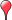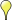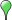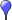# Portugal Weather Network

## PTWN

 Temperature [ C° ] Dew Point [ C° ] Humidity [ % ] Wind [ km/h ] Rain Today [ mm ] Pressure [ hPa ] Fire Danger [Chandler Burning Index] °C °F km/h mph m/s kts mm in hPa inHg mb

[] Weather, WebCam, Lightning, [] Weather, Lightning, [] Weather, WebCam, [] Weather

Conditions data shown was collected
from Sat, 25-Jan-316889355 17:46:39 to Thu, 01-Jan-1970 01:00:00

Regional mesonet-map script by Saratoga-Weather.org

### Current Conditions

Conditions data shown was collected
from Sat, 25-Jan-316889355 17:46:39 to Thu, 01-Jan-1970 01:00:00

Note: Click on the table column title to change the order of that column's vaues.

Area

Station
Current
Cond.
Temp.
°C
Dew Point
°C
Humid
%
Wind
km/h
Gust
km/h
Rain
mm
Pressure
hPa
Trend Fire
Danger
Last
Update

### Stations Features

• "IP 85.233.160.31 has been unblocked"
• [ ]
• \$("#text").text(
• [ ]
• [ ]
• [ ]
• \$('.dropdown-title').text(currentLocaleName);
• [ ]
• \$('.wraper').show(); // show our text and stuff
• [ ]
• \$([].find.call(\$('.dropdown-menu li'), function (item) {
• [ ]
• [ ]
• \$.ajax({
• [ ]
• [ ]
• [ ]
• (function () {
• [ ]
• (function checkVisibility () {
• [ ]
• (function update () {
• [ ]
• );
• [ ]
• * (captcha is shown to user).
• [ ]
• [ ]
• * 2. display some additional text
• [ ]
• * 3. display a dropdown so user can switch to a different language
• [ ]
• * Detecting captcha when css is changed to visible.
• [ ]
• * Invisible reCaptcha has no API calls when captcha will be visible,
• [ ]
• * When captcha changed css "visibility" to the "visible", we should:
• [ ]
• */
• [ ]
• -->
• [ ]
• [ ]
• -moz-transform: rotate(0deg);
• [ ]
• -moz-transform: rotate(360deg);
• [ ]
• -ms-transform: rotate(0deg);
• [ ]
• -ms-transform: rotate(360deg);
• [ ]
• -o-transform: rotate(0deg);
• [ ]
• -o-transform: rotate(360deg);
• [ ]
• -webkit-animation: spin 2s ease-in-out infinite;
• [ ]
• -webkit-transform: rotate(0deg);
• [ ]
• -webkit-transform: rotate(360deg);
• [ ]
• .append(Object.keys(items).sort().map(function (item) {
• [ ]
• [ ]
• .bottom {
• [ ]
• [ ]
• .cap_mess {
• [ ]
• .cap_side {
• [ ]
• .cap_text {
• [ ]
• [ ]
• [ ]
• [ ]
• .click(function (e) {
• [ ]
• [ ]
• .dropdown .dropdown-menu-content > li:hover {
• [ ]
• [ ]
• .dropdown .dropdown-toggle {
• [ ]
• .dropdown .dropdown-toggle:hover {
• [ ]
• .dropdown .glyphicon {
• [ ]
• .dropdown li {
• [ ]
• .dropdown li.active {
• [ ]
• .dropdown li:focus, .dropdown li:hover {
• [ ]
• .dropdown li:last-child {
• [ ]
• .dropdown {
• [ ]
• .dropdown.open .glyphicon {
• [ ]
• [ ]
• [ ]
• [ ]
• .invisible_mode .wraper {
• [ ]
• .logo_shield img {
• [ ]
• .logo_shield {
• [ ]
• .middle {
• [ ]
• .powered_span {
• [ ]
• .rot {
• [ ]
• .visible_mode #re-captcha { /* align to center */
• [ ]
• [ ]
• .wraper {
• [ ]
• /* A few hacks for re-captcha */
• [ ]
• [ ]
• /**
• [ ]
• // add class to reCAPTCHA wrapper so our css would apply to it
• [ ]
• // change the size of our block to fit the captcha block
• [ ]
• [ ]
• //this will reload the page, it's likely better to store this until finished
• [ ]
• 0% {
• [ ]
• 100% {
• [ ]
• [ ]
• [ ]
• [ ]
• [ ]
• [ ]
• [ ]
• [ ]
• [ ]
• [ ]
• [ ]
• [ ]
• [ ]
• [ ]
• [ ]
• [ ]
• [ ]
• [ ]
• [ ]
• [ ]
• [ ]
• [ ]
• [ ]
• [ ]
• [ ]
• [ ]
• [ ]
• [ ]
• [ ]
• [ ]
• [ ]
• [ ]
• [ ]
• [ ]
• [ ]
• [ ]
• [ ]
• [ ]
• [ ]
• Please confirm that you are not a robot
• [ ]
• [ ]
• [ ]
• [ ]
• [ ]
• [ ]
• [ ]
• [ ]
• [ ]
• [ ]
• [ ]
• [ ]
• [ ]
• [ ]
• [ ]
• [ ]
• [ ]
• [ ]
• [ ]
• #### www.meteoalentejo.pt

• [ ]
• [ ]
• [ ]
•• [ ]
• [ ]
• [ ]
• [ ]
• [ ]
• [ ]
• [ ]
• [ ]
• [ ]
• [ ]
• [ ]
• [ ]
• [ ]
• [ ]
• [ ]
• [ ]
• [ ]
• [ ]
• [ ]
• [ ]
• [ ]
• [ ]